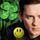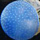## Social QuestionAsked by LostInParadise (29137) October 2nd, 2020
6 responses
“Great Question” (1)

The psychologist Dan Kahneman talks about what he calls System 1 and System 2 thinking. System 1 is intuitive and quick and System 2 is more thoughtful but slower.

One example he gives of System 1 taking over is the following problem. A bat and ball together cost \$1.10. If the bat costs a dollar more, what is the price of the ball? Kahneman says that the answer that most people come to immediately is that the ball costs 10 cents. Many will give the answer without even testing it, which would show immediately that it is wrong. Ten cents + \$1.10 is \$1.20, not \$1.10.

The problem is easily solved with elementary algebra, but is there an intuitive non-algebraic approach? Suppose we start with both items being the same price, 55 cents. If we add an amount to the first and subtract the same amount from the second, the total will remain \$1.10. Furthermore, the difference between the two prices will be twice the amount added and subtracted. Therefore, if the common amount is 50 cents, we will end up with a difference of \$1. So the ball costs 55 cents minus 50 cents equals 5 cents.

This type of calculation comes up a lot when you hear about voting. If one person gets 5% more of the vote than the other person, how much of the vote did they each get. We start with 50% each and add and subtract 5/2% = 2.5% to get 52.5% and 47.5%.Follow this questionSend to a friend!Topics: ,
Observing members: 0Composing members: 0Dividing the sum by 2 and then adding a surtracting the same amount works really well in those relatively easy examples. Would that method be called System 1.5?
It is a little slower than the intuitive System 1 but not as slow and thoughtful as System 2.

LuckyGuy (40159)“Great Answer” (1)Kahneman might answer that with enough practice it might become a part of System 1. A lot of activities like learning to play a sport, start out as System 2 but eventually get incorporated into System 1.

LostInParadise (29137)“Great Answer” (0)Before th days of calculators. I used to do square roots using System 1

Example. What is the square root of 100? Guess at a value reasonably close. ( We know tha answer is 10 but let’s guess 9) Divide 9 into 100 = 11.1 . Then average 9 and 11 to get = 10.05 The closer your guess, the closer your result .
Two iterations and you are within 1%.

LuckyGuy (40159)“Great Answer” (2)I have seen that method for calculating square root. It is a specific example of Newton’s method.

If I remember right, Newton’s method for finding the kth root of n is to take a guess x and then get a value y = n/x^(k-1). The next estimate is (k-1)/k x + 1/k y. One way of thinking about it is to say that if x is a little bit off, raising x to the (k-1) power is going to be off by a lot more, so you give greater weight to x than to y.

LostInParadise (29137)“Great Answer” (1)Your method is not an “intuitive algebraic” method, it is an iterative calculation.

doyendroll (1771)“Great Answer” (2)@LuckyGuy , It is pretty neat that you found that method on your own. If you look at the diagram for Newton’s method, you will see that it also has a nice intuitive feel to it.

LostInParadise (29137)“Great Answer” (1)